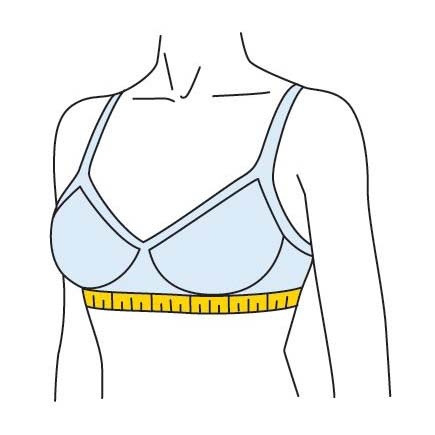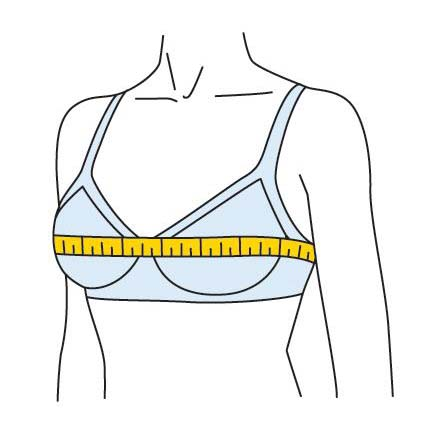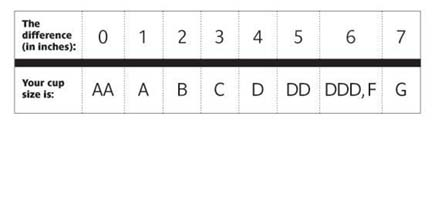# How To Find The Bra Size of a Sex Doll### Step 1 - Determine Your Band Size

1. Measure around the torso just under the breasts.  Make sure the measuring tape is very snug and level around the body.
2. Round the measurement to the next highest whole number.
3. If the number is even + 4", if the number is odd + 5".

Even Measurement Example:

• Torso circumference measurement right below breasts = 25.98"
• Round Up: = 26"  + 4" = 30"
• The Band Size is 30

Odd Measurement Example:

• Torso circumference measurement right below breasts = 26.77"
• Round Up: = 27"  + 5" = 32"
• The Band Size is 32### Step 2 - Get Bust Measurement

1. Wrap tape measurement loosely around the fullest part of the bust (at nipple level).
2. Round to up to the next highest whole number.### Step 3 - Determine Cup Size

Subtract the band size from the bust measurement and refer to the chart.

Example

• Bust measurement 33" - Band size 30"  = 3"
• Difference of 3" = C cup